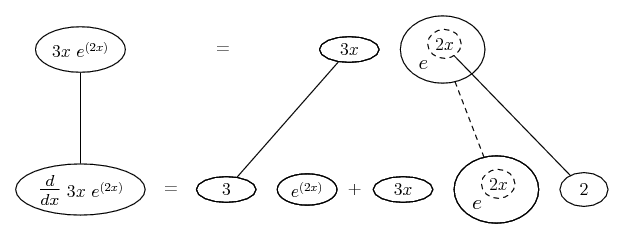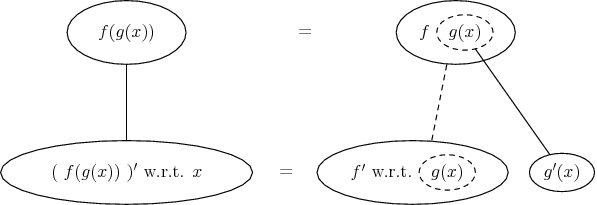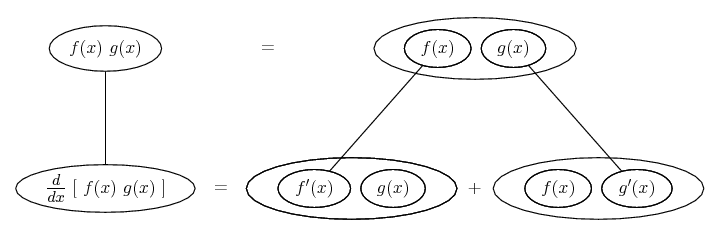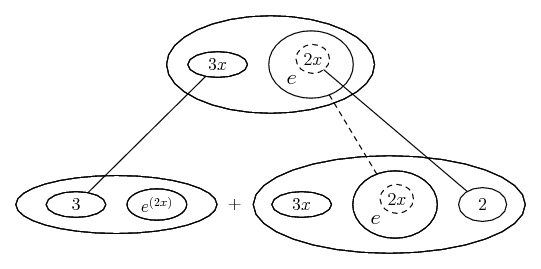# Thread: Derivatives - product rule with three terms?

1. ## Derivatives - product rule with three terms?

I'm trying to get the derivative of 3xe^(2x)

I know the product rule and I know the derivative of e^2x is 2e^(2x).
So I was going to use the product rule, but I've only ever done that with two terms. Here, there are three.

2. You have a function $\displaystyle h(x)=f(x)g(x)$ where $\displaystyle f(x)=3x$ and $\displaystyle g(x)=e^{2x}$

$\displaystyle h'(x)=f'(x)g(x)+f(x)g'(x)$

$\displaystyle h'(x)=(3)e^{2x}+3x(2e^{2x})$

$\displaystyle h'(x)=3e^{2x}+6xe^{2x}$

3. the 3 is just a constant, BUT if you have three functions then

$\displaystyle (fgh)'=f'gh+fg'h+fgh'$.

4. thats a product rule and a chain rule

3xe^(2x)

3e^(2x)

for the first. take derivative of 3x which is just 3 and keep second part. than. chain rule e^(2x) so e^ whatever is just e^ the whatever. so e^(2x) than multiply by 2 since the derivative of 2x is 2. keep the first part.

So you get:
3e^(2x) + 3xe^(2x)*2 which is 3e^(2x) + 6xe^(2x)
you can then simplify by pulling 3e^(2x) out of the answer
3e^(2x)*(1+2x) but that wouldnt really help unless your graphing and looking for critical numbers

5. Just in case a picture helps...You have the chain rule - generally this pattern -... wrapped inside the product rule...... more visible here if we enclose some of the balloons in some more...Hope this helps, or doesn't further confuse.
Don't integrate - balloontegrate!
Balloon Calculus: worked examples from past papers

,
,

,

,

,

,

,

,

,

# How to differentiate three or more functions using product rule

Click on a term to search for related topics.

#### Search Tags

derivatives, product, rule, terms